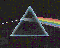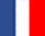# Exact sign of determinant computation

This is a work of F. Avnaim, J-D. Boissonnat, O. Devillers, F. Preparata, and M. Yvinec.

We propose a method to evaluate signs of 2x2 and 3x3 determinants with b-bit integer entries using \$b\$ and (b+2)-bit arithmetic respectively. This algorithm has numerous applications in geometric computation and provides a general and practical approach to robustness. The algorithm has been implemented and experimental results show that it slows down the computing time by only a small factor with respect to floating-point calculation, and compare favorably with other exact methods.

The main practical interest of our method, compared to other exact evaluation methods, is that it allows to use the very fast standard arithmetic of the computer while it does not assume a too small range of the original data.

Practically, using the 53 bits IEEE standard 754 arithmetic for provided for double allows to compute exactly the sign of 2x2 determinants with 53 bits integer entries and 3x3 determinants with 51 bits integer entries.

Software.

 Last modified: Thu Nov 25 14:17:26 MET 1999 Olivier Devillers Software